# ML Aggarwal Class 7 Solutions for ICSE Maths Chapter 7 Percentage and Its Applications Check Your Progress

## ML Aggarwal Class 7 Solutions for ICSE Maths Chapter 7 Percentage and Its applications Check Your Progress

Question 1.
Convert the following percentages into fractions in the simplest form:
(i) 12$$\frac { 1 }{ 2 }$$ %
(ii) 66$$\frac { 2 }{ 3 }$$ %
(iii) 8$$\frac { 1 }{ 3 }$$ %
Solution:Question 2.
Express each of the following fractions as a percentage:
(i) $$\frac { 5 }{ 8 }$$
(ii) $$\frac { 13 }{ 40 }$$
(iii) $$\frac { 7 }{ 6 }$$
Solution:Question 3.
Express each of the following percentages as a decimal:
(i) 122%
(ii) 2.2%
(iii) 3$$\frac { 1 }{ 8 }$$ %
Solution:Question 4.
Express 0.0345 as a percentage.
Solution: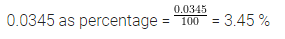Question 5.
Convert each part of the ratio 5 : 6 : 9 to a percentage.
Solution: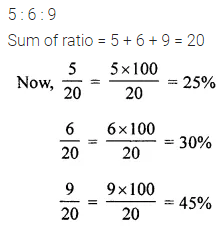Question 6.
(i) What percent of a day is half an hour?
(ii) What percent is $$\frac { 3 }{ 4 }$$ metres of 4$$\frac { 1 }{ 2 }$$ metres?
Solution: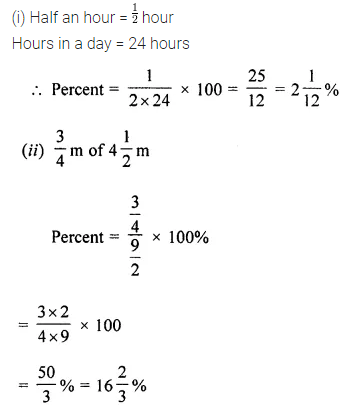Question 7.
The population of a town decreased from 25000 to 24500. Find the percentage decrease.
Solution: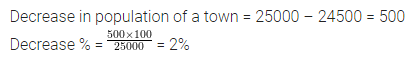Question 8.
Arun bought a car for ₹ 350000. The next year, the price went upto ₹ 370000. What was the precentage increase in the price?
SolutionQuestion 9.
The population of a village has decreased by 6%. If the original population was 3650, find the population after decrease.
Solution: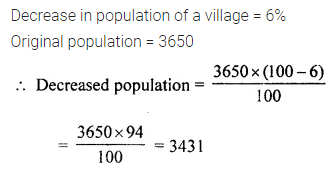Question 10.
43% of the students in a school are girls. If the number of boys is 1482, find:
(i) the total strength of the school
(ii) number of girls in the school.
Solution: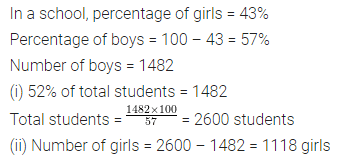Question 11.
Rohan bought a calculator for ₹ 760 and sold it for ₹ 874. Find his profit and profit percentage.
Solution: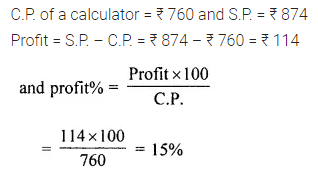Question 12.
On selling an article for ₹ 1027, Meena suffered a loss of ₹ 273. Find her loss percentage.
Solution: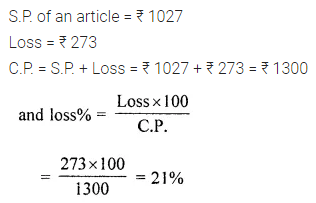Question 13.
By selling a fan for ₹ 710, a trader suffers a loss of ₹ 40. Find the cost price of the fan. At what price this fan should be sold in order to gain 10%?
Solution:Question 14.
A shopkeeper sells an article at ₹ 300, thus earning a profit of 20%. Find the cost price of the article.
Solution: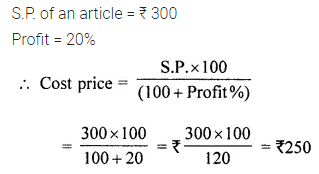Question 15.
A shopkeeper sells an article at ₹ 320, thus suffering a loss of 20%. Find the cost price of the article.
Solution: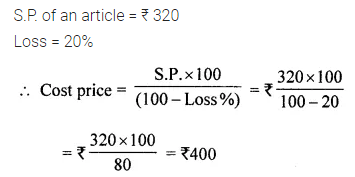Question 16.
If ₹ 6000 is borrowed at 6.5% per annum simple interest, find the interest and the amount to be paid at the end of 3 years.
Solution: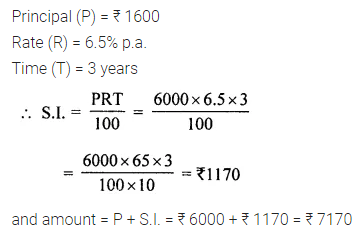Question 17.
How long will it take for ₹ 1860 invested at the rate of 9.5% per annum simple interest to amount to ₹ 2449?
Solution:Question 18.
At what rate will ₹ 7200 fetch a simple interest of ₹ 3024 in 4 years?
Solution: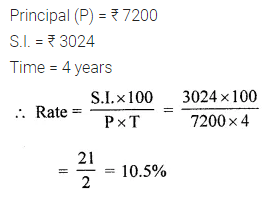Question 19.
What sum of money will yield a simple interest of ₹ 1155in 3 years 6 months at 11% p.a.?
Solution: